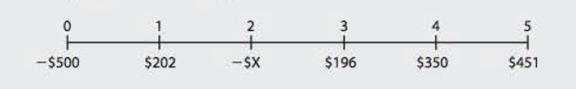Chapter 11, Problem 22PFundamentals of Financial Manageme...

14th Edition
Eugene F. Brigham + 1 other
ISBN: 9781285867977

Solutions

Chapter
SectionFundamentals of Financial Manageme...

14th Edition
Eugene F. Brigham + 1 other
ISBN: 9781285867977
Textbook Problem

MIRR A project has the following cash flows:This project requires two outflows at Years 0 and 2, but the remaining cash flows are positive. Its WACC is 10%, and its MIRR is 14.14%. What is the Year 2 cash outflow?

Summary Introduction

To compute: The Year 2 cash outflow.

Introduction:

Modified Internal Rate of Return (MIRR): It refers to the rate of return that is computed by the company to make a decision of selection and ranking of a project for investment. This is a modified version of IRR with reinvestment of cash flows at the cost of capital.

Explanation

Given information:

Initial investment of project is $500. Cash inflow at the end of year 1 is$202.

Cash inflow at the end of year 3 is $196. Cash inflow at the end of year 4 is$350.

Cash flow at the end of year 5 is $451. WACC of the project is 10%. MIRR of the project is 14.14%. Terminal value is$1,369 (working note).

The formula to calculate MIRR is,

MIRR=(TerminalValuePresentValue Costs)1N1

Where,

• N is the number of years.

Substitute $1,369 for terminal value, 14.14% for MIRR, ($500+$X) for present value costs and 5 for N in the above formula. 0.1414=($1,369($500+$X))151(1.1414)5=($1,369$500+$X)1.9373=$1,369$500+$X$500+$X=\$706

Still sussing out bartleby?

Check out a sample textbook solution.

See a sample solution

The Solution to Your Study Problems

Bartleby provides explanations to thousands of textbook problems written by our experts, many with advanced degrees!

Get Started

Find more solutions based on key concepts